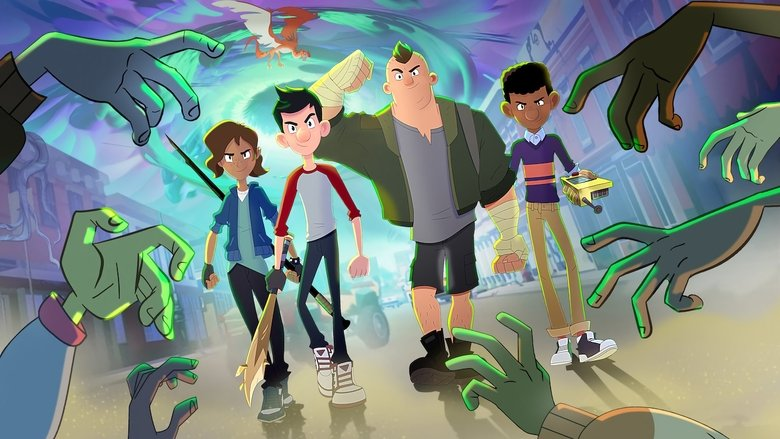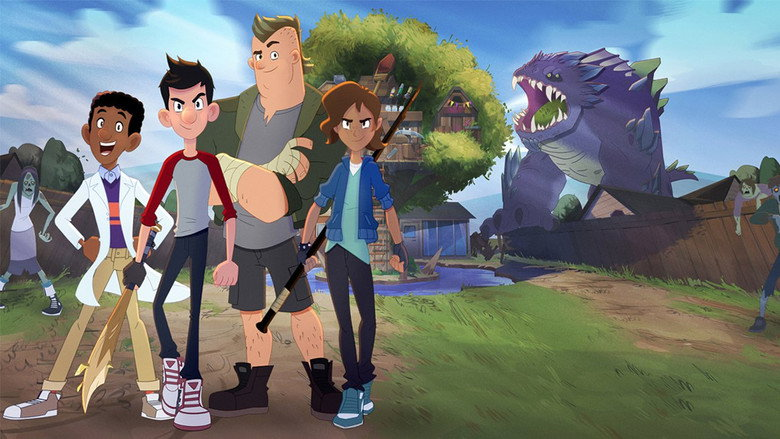{{{ tweet.body | format }}}

×

# Benachrichtigungen• Regie
• Drehbuch
• Cast1x1 – Folge 1 The Kids Battle The Zombified Creatures. 1x2 – Mall Quest Know what this is about? Be the first one to add a plot. 1x3 – Jack the Slayer Know what this is about? Be the first one to add a plot. 1x4 – Bestiary Master Know what this is about? Be the first one to add a plot. 1x5 – The Zombie Parade Know what this is about? Be the first one to add a plot. 1x6 – The Thrull of Victory Know what this is about? Be the first one to add a plot. 1x7 – Stay on Target Know what this is about? Be the first one to add a plot. 1x8 – June Gloom Know what this is about? Be the first one to add a plot. 1x9 – Follow That Butler Know what this is about? Be the first one to add a plot. 1x10 – Always Darkest Know what this is about? Be the first one to add a plot. 1x11 – Dawn of Rezzoch Know what this is about? Be the first one to add a plot.
••Quelle: themoviedb.org

Es liegt noch keine offizielle Kritik für diese Serie vor.